# Finding unknown values in DC resistor network via matrices

• Dethoven
A: -1.6815, 0.5451, 0.0358B: 0.4609, -0.8536, 0.1492C: 0.3912, -0.7476, 0.1498D: 0.2969, -0.5697, 0.1492E: 0.2248, -0.4696, 0.1492Yes, this is the matrix I solved. The result was that the device was OFF.f

## Homework Statement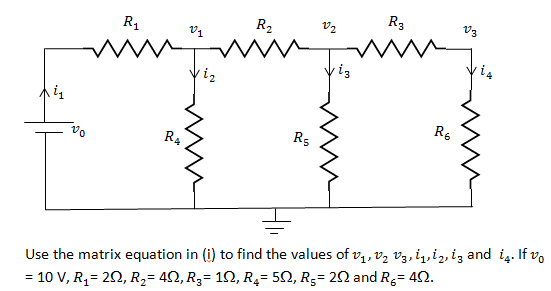I need to get 7 equations to make a 7x7 matrix in order to solve it. I have 4 of them, but I'm not sure how to get the voltages equations.

## The Attempt at a Solution

I have got four equations so far. One is simply using KCL, I1 = I2 + I3 + I4. The other three are from using KVL and Ohm's Law to get:
1. V0 - VR1 - VR4 = 0
2. VR4 - VR2 - VR5 = 0
3.VR5 - VR3 - VR6 = 0

Applying Ohm's Law:
1. V0 - R1i1 - R4i2 = 0
2. R4i2 - R2(i3 + i4) - R5i3 = 0
3. R5i3 - R3i4 - R6i4 = 0

How do I get 3 equations for the unknown voltages?

I need to get 7 equations to make a 7x7 matrix in order to solve it.
Are you asked to use this specific method? There are much simpler methods to solve this circuit.

Are you asked to use this specific method? There are much simpler methods to solve this circuit.
Yes, I will be using MATLAB to solve it.

using your 3 equations you can solve for the currents. Once you know the currents you should know the voltages.
V=IR

Once you know all the currents in a circuit, it should be easy to find all the voltages

Yes, I will be using MATLAB to solve it.
Ok, but then also a 3×3 matrix will be sufficient if you used mesh analysis.

I suspect that the OP is meant to use a more general matrix method involving network theory and incidence matrices,etc. But he hasn't spelled this out, nor provided the relevant equations so that we can confirm this or help in a meaningful way. The mention of a 7 x 7 matrix is what gives me this feeling.

I suspect that the OP is meant to use a more general matrix method involving network theory and incidence matrices,etc. But he hasn't spelled this out, nor provided the relevant equations so that we can confirm this or help in a meaningful way. The mention of a 7 x 7 matrix is what gives me this feeling.
I apologise for the incomplete question, I admit I was in a rush typing it out. Here is the relevant question:

You are given V0, and the values of six resistors. The seven unknowns can be treated as elements of a 7x1 matrix. Using Kirchoff's Law and Ohm's Law, write the solution to the resistor network as a matrix equation containing a 7x7 matrix.

Essentially, I will have A*X = B where I'm solving for X.

Last edited:
Oh my, if I simply rewrite each resistor as equations in the 7 unknowns, for example, for R1: R1I1 = V0 - V1, then make V0 the subject, will that be fine? And for the 7th equation, I use I1 = I2 + I3 + I4?

Oh my, if I simply rewrite each resistor as equations in the 7 unknowns, for example, for R1: R1I1 = V0 - V1, then make V0 the subject, will that be fine? And for the 7th equation, I use I1 = I2 + I3 + I4?
see my post above.

If you have equations for the currents you should be able to rework them for the voltage equations

see my post above.

If you have equations for the currents you should be able to rework them for the voltage equations
But I need 7 equations, from which I can form a matrix and solve.

But I need 7 equations, from which I can form a matrix and solve.

AX=B,
you're solving for X

A is a 7x7
B is a 7x1
X is a 7x1

so B(1)=A(1,1)*X(1) + A(2,1)*x(1)... etc
lets say x = [i1 i2 i3 i4 v1 v2 v3]'

you should be able to find equations for v1 v2 and v3 and fill in the A matrix accordingly. You're really telling me you can't find a relationship between current and voltage across a given resistor...

If you want to form equations for the node voltages I suggest you use nodal analysis (KCL) at each of the nodes. You found the current equations using KVL around the loops, so similarly you can find node voltage equations using KCL at the nodes.

I've now got 6 equations, which are hopefully right. What would be the 7th equation?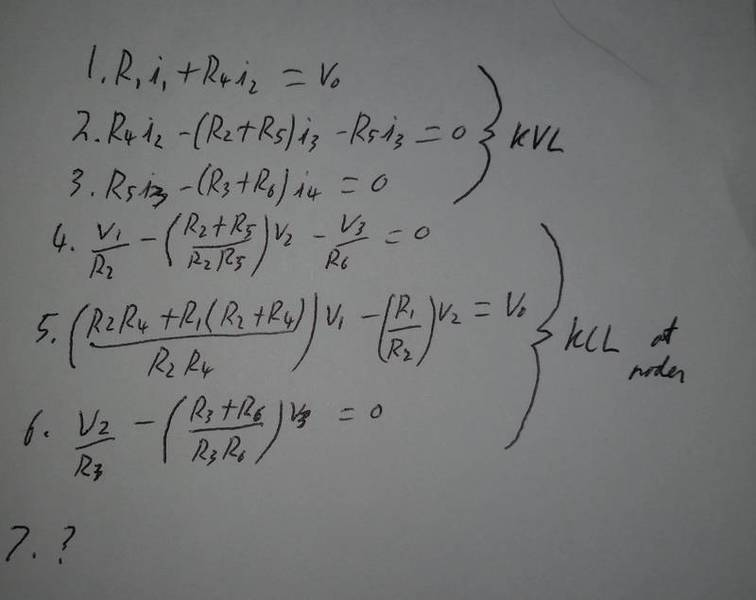How about the sum of all the currents? What's the relationship between i1 and the rest of the currents?

Do you know the correct values for the currents and voltages?

Have you been able to solve your 7 equations? Did that solution give you the correct values?

The reason I ask is because I solved your equations and didn't get the correct values for the currents.

I added the equation suggested by gneill as equation 4 and get this as the 7x7 system you're trying to solve: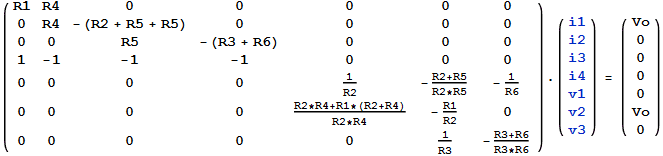Is this the matrix you solved? What was the result?

I get this, and the currents are not correct: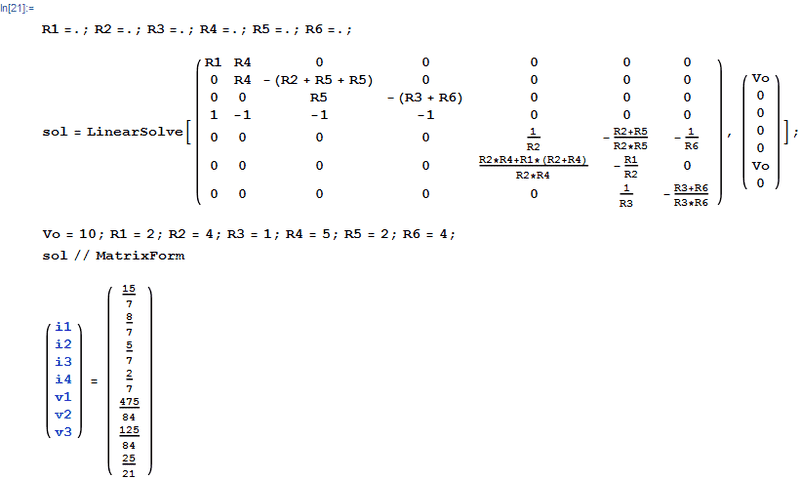I've now got 6 equations, which are hopefully right. What would be the 7th equation?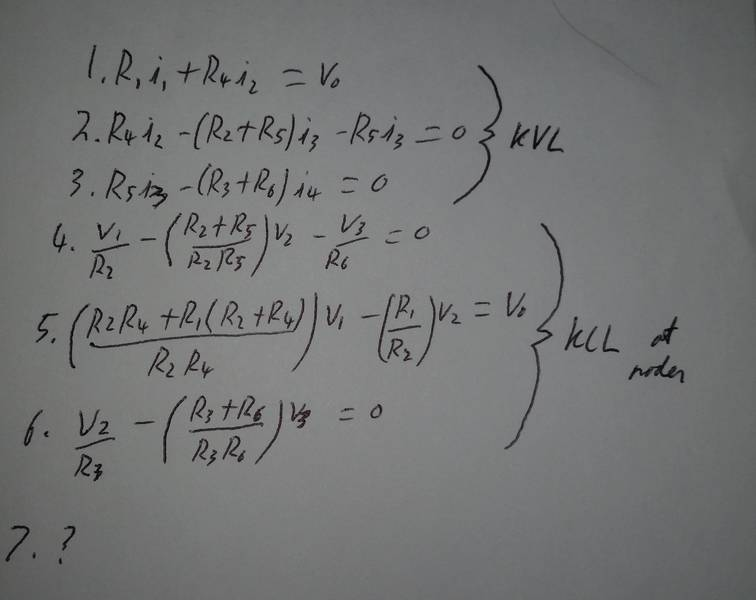why don't you use ohms law to calculate the voltages?
i2*R4=V1
etc
thats three easy equations
and the equation recommended by gneil.

Do you know the correct values for the currents and voltages?

Have you been able to solve your 7 equations? Did that solution give you the correct values?

The reason I ask is because I solved your equations and didn't get the correct values for the currents.

I added the equation suggested by gneill as equation 4 and get this as the 7x7 system you're trying to solve:

View attachment 99535

Is this the matrix you solved? What was the result?

I get this, and the currents are not correct:

View attachment 99537